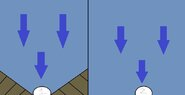# Question regarding water pressure and slopes

• Jstoff
In summary, the conversation discusses the effects of shape and surrounding environment on water pressure PSI readings. After further clarification, it is concluded that both sensors would read the same value of pressure, but the flow rate through an orifice can vary depending on its shape. The concept of Pascal's law is also mentioned as a possible explanation for the scenario.

#### Jstoff

TL;DR Summary
Questioning regarding water pressure PSI and slopes.
I have a question about water pressure PSI regarding the presents/lack of slopes.

If two identical pressure sensors are set up on the ocean floor at the same depth. One being surrounded by a cone of ramps increasing in height moving outwards away from it. The second sitting on a flat plain with no increase or decrease of the surrounding ocean floor. (I've attached an illustration for explain.) In this situation would the sensors read the same or different PSI? If different which one would be higher?

Sorry if this isn't the correct area to post in. Wasn't sure what this fit under honestly. Thanks for the help!

#### Attachments

•Waterpressure.jpg
11.8 KB · Views: 85
Lnewqban said:
Welcome!
Both sensors will read the same value of pressure.

https://www.physicsforums.com/threa...-depend-on-the-shape-of-the-container.978355/
I appreciate the quick reply! Thanks for the link I believe it really cleared things up for me. It also made me relies that i forgot an important part of my question. To be honestly I'm not sure what measurement would be most appropriate for the answer of this question. I believe its head pressure but I'm not sure if I'm using it in the right context.

What if sensors 1 and 2 were replaced with twos holes of the exact same dimensions. On the other side of the hole there's a large empty container. Would the force of water coming out the other side be the same? I'm assuming they would if the static water's PSI was the same. Just i just wanted to make sure.

•256bits
You understand that the static pressure (with nothing moving) is always the same.
The flow rate through an orifice depends upon more things than just the pressure. Our ability to describe such situations simply is very limited although it has been the subject of intense scrutiny for as long as there has been science. Now we have powerful computing machines and it is still difficult. In previous decades and centuries various specific and extraordinarilly clever approximations in fluid dynamics have been developed.
In general a more "streamlined" (a term from said fluid dynamics) orifice will produce more flow.

•256bits
Jstoff said:
... What if sensors 1 and 2 were replaced with twos holes of the exact same dimensions. On the other side of the hole there's a large empty container. Would the force of water coming out the other side be the same? I'm assuming they would if the static water's PSI was the same. Just i just wanted to make sure.
I can’t visualize what you describe, but Pascal’s law could be applied to that case:

https://en.m.wikipedia.org/wiki/Hydrostatics

Jstoff said:
Would the force of water coming out the other side be the same?
It's not a force that come out, but it is quantified as flow rate, either volumetric or mass(emetric), momentum, velocity. The force comes about only when the stream contacts another surface and should not be confused with the above.

•Lnewqban and hutchphd

## 1. What is water pressure?

Water pressure is the force exerted by water on the walls of a container or surface. It is caused by the weight of the water above the surface and is measured in units of pressure, such as pounds per square inch (psi) or pascals (Pa).

## 2. How does water pressure change with slope?

Water pressure increases with slope. As water flows down a slope, the force of gravity pulls it downward, increasing the weight of the water and therefore increasing the pressure on the surface below.

## 3. What is the relationship between water pressure and depth?

Water pressure increases with depth. As the depth of water increases, there is more water above the surface, increasing the weight and therefore the pressure.

## 4. How does water pressure affect structures on slopes?

Water pressure can be a significant factor in the stability of structures on slopes. If the water pressure becomes too high, it can cause erosion and destabilize the structure. It is important to consider water pressure when designing and constructing structures on slopes.

## 5. How can water pressure be measured?

Water pressure can be measured using a pressure gauge or a manometer, which measures the height of a column of water. It can also be calculated using the equation P = ρgh, where P is pressure, ρ is the density of water, g is the acceleration due to gravity, and h is the height of the water column.